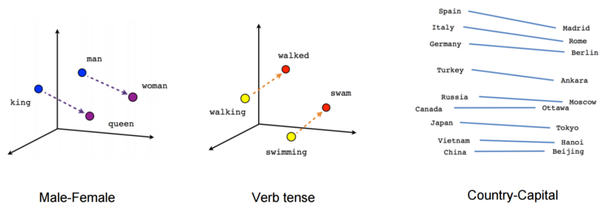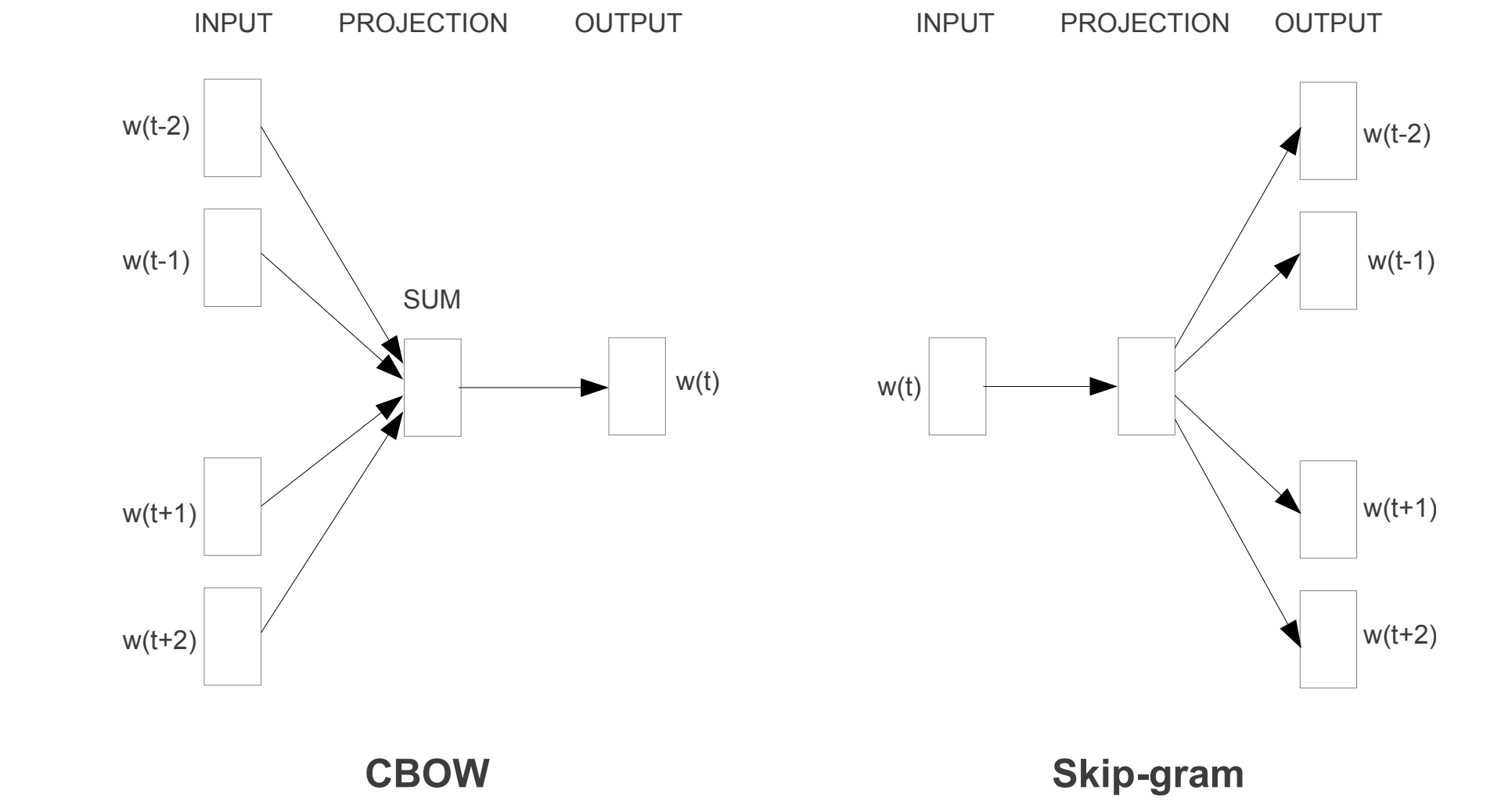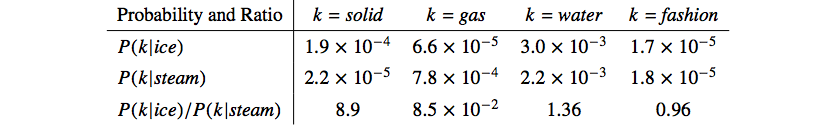# Word Embeddings## 1. Why Embedding?

Many classic NLP systems and techniques (i.e. popular N-gram model) treat words as atomic units. There is no notion of similarity between words, as they are represented as indices in a vocabulary. This approach works when trained on huge amounts of data, but are limited in many tasks when data is limited (i.e. speech recognition data, parallel corpus for machine translation). Learning representation of words establishes similarity between words and allows data to be used much more efficiently.

## 2. word2vec

The high-level assumption of word2vec is that words close to each other have more similar meaning than words far apart. Under this assumption, two model architectures were proposed by the researchers at Google.### 2.1 Skip-gram Model

The objective of the Skip-gram model is to find word representations that are useful for predicting the surrounding words in a sentence or a document. The objective of the model is to maximize the average log probability

$$\frac{1}{T} \sum_{t=1}^{T} \sum_{-c \leq j \leq c, j \neq 0} \text{log} \ p(w_{t+j}|w_{t})\tag{1}$$

The probability $p(w_{t+j}|w_{t})$ is defined using the softmax function:

$$p(w_{O}|w_{I}) = \frac{\text{exp} ( u_{w_{O}}^T v_{w_{I}})}{\sum_{w=1}^W \text{exp} ( u_{w}^T v_{w_{I}})}\tag{2}$$

where $v$ and $u$ are input and output representation of the word. This is impractical since computing the gradient w.r.t the entire vocabulary is $\mathcal{O}(W)$, the size of the vocabulary.

#### Hierarchical Softmax

A computationally efficient approximation of the full softmax is hierarchical softmax, which uses a binary tree representation of the output layer with $W$ words as its leaves. With the words as leaf nodes, then we only need to follow the path to the leaf node of that word, without having to consider any of the other nodes. $p(w|w_I)$ simply the product of the probabilities of taking right and left turns respectively that lead to its leaf node. This is much faster since computing the gradient is $\mathcal{O}( \text{log} \ W)$.

#### Negative Sampling

We can also subsample the entire vocabulary to compute the softmax. The idea of Noise Contrastive Estimation (NCE) is to train binary logistic regressions for a true pair (center word and word in its context window) vs a couple of noise pairs (the center word paired with a random word). The objective with NCE is

$$\text{log} \ \sigma( u_{w_{O}}^T v_{w_{I}}) + \sum_{j \sim P(w)} \text{log} \ \sigma( - u_{w_{j}}^T v_{w_{I}})\tag{3}$$

The intuition of the above loss is to maximize the probability that real context word appears through the first term and minimize probability that random words appear around center word through the second term.

The noise distribution $P(w) = U(w)^{¾}/Z$, where $U(w)$ is the unigram distribution.

#### Subsampling Frequent Words

To counter the imbalance between the rare and frequent words, each word $w_i$ in the training set is discarded with probability of $P(w_i) = 1 - \sqrt{\frac{t}{f(w_i)}}$, where $f(w_i)$ is the frequency of the word and $t$ is a chosen threshold with typical value of $10^{-5}$. For words whose frequency is greater than t, it is subsampled aggressively.

### 2.2 Continuous Bag-of-Words (CBOW)

CBOW is a similar idea to Skip-gram, but instead of predicting the context words based on current word, it predicts the current word based on the context. The model is named bag-of-words since the order of words in the context does not influence the model, where an average of the context word vectors are used to predict the current word. Same as Skip-gram, we seek to maximize the average log probability

$$\frac{1}{T} \sum_{t=1}^{T} \text{log} \ p(w_{t}|\bar{w_{t}})\tag{4}$$ where the input vector is the average of all context word vectors $\bar{w_{t}} = \frac{1}{2c}\sum_{-c \leq j \leq c, j \neq 0} w_{t+j}$. Different from the Skip-gram model, the weight matrix of input and output word vectors is shared. The authors found that CBOW

• Slightly outperforms on the Syntactic Accuracy tests (“apparent” -> “apparently” = “rapid” -> “rapidly”)
• Underperforms significantly on Semantic Accuracy tests (“Athens” -> “Greece” = “Oslo” -> “Norway”)

## 3. GloVe

About a year after word2vec was published, Pennington et al. from Stanford came up with a new global model that combines the advantages of global matrix factorization methods (i.e. LSA) and local context window methods (i.e. word2vec).

#### Matrix Factorization vs Local Context Windows

Matrix factorization methods for generating low-dimensional word representations utilize low-rank approximations to decompose large matrices that capture statistical information about a corpus. In LSA, the matrix is term-document matrix filled with counts of words in each documents. In Hyperspace Analogue to Language (HAL), the matrix is a term-term matrix filled with counts of words co-occurring in the same context.

The main problem with Matrix Factorization Methods is that the most frequent words contribute a disproportionate amount to the similarity measurement, despite conveying relatively little semantic relatedness. The local context window models, on the other hand, suffer from the disadvantage that they do not operate directly on the co-occurrence statistics of the corpus.

#### Deriving the GloVe Model

Let $X$ be the word-word co-occurrence count matrix, where $X_{ij}$ denote the number of times word $j$ occurs in the context of word $i$. $X_i = \sum{k} X_{ik}$ is the number of times any word appears in the context of word $i$. Let $P_{ij} = P(j|i) = X_{ij} / X_i$ be the probability that word $j$ appear in the context of word $i$.

GloVe originates from the idea that meaning can be extracted directly from co-occurrence probabilities. Consider two words i = ice and j = steam; we see that $P_{ik}/P_{jk}$ is large when $k$ is related to $i$, but not $j$ and vice versa. When $k$ is either related to both or unrelated to both, $P_{ik}/P_{jk}$ is close to 1.The above argument suggest that the word vector should be learned with ratios of co-occurrence probabilities. The most general model can be in the form of

$$F\left( w_i, w_j, \tilde{w}_k \right) = \frac{P_{ik}}{P_{jk}}\tag{5}$$

We can restrict $F$ in the following way

• $F$ should be only dependent on the difference of the two target words $w_i - w_j$
• To keep the linear nature of the vector space, restrict $F$ to take the dot product of the arguments $(w_i - w_j)^T \tilde{w}_k$
• By requiring the role of target words and context words can be exchanged, we have $F\left((w_i - w_j)^T \tilde{w}_k \right) = F(w_{i}^T\tilde{w}_{k}) / F(w_{j}^T\tilde{w}_{k})$

This gives us the solution of $F = \text{exp}$ and $w_{i}^T\tilde{w}_{k} = \text{log} (P_{ik}) = \text{log} (X_{ik}) - \text{log} (X_{i})$. To restore the symmetry between target word and context words, we can set $b_i=\text{log} (X_{i})$ and add an bias term $\tilde{b}_k$,

$$w_{i}^T\tilde{w}_{k} + b_i + \tilde{b}_k = \text{log} (X_{ik})\tag{6}$$

A main drawback of this model is that it weighs all co-occurrence equally. To fix this problem, we add weights to the model. The weighted least squares regression model has the following cost function:

$$J = \sum_{i,j=1}^{V} f(X_{ij}) \left( w_{i}^T\tilde{w}_{k} + b_i + \tilde{b}_j - \text{log} (X_{ij}) \right)^2 \tag{7}$$

where $f(x)$ is defined very similar to $P(w)$ from word2vec.

$$f(x) = \begin{cases} (x/x_{max})^{¾}, & x < x_{max} \\ 1, & \text{otherwise} \end{cases}$$

#### Context Window

GloVe shares the same assumption that very distant word pairs are expected to contain less relevant information about the words’ relationship to one another. Skip-gram defined “context” as a moving window. GloVe specifies that word pairs that are $d$ words apart contribute $1/d$ to the total count. Hence Global vectors to account for context.

#### Relationship to Skip-gram

Let $Q_{ij}$ be the softmax and $X_{ij}$ be the context co-occurrence, the objective of the skip-gram function is

$$J = - \sum_{i}\sum_{j \in context(i)} \text{log} (Q_{ij}) = - \sum_{i}\sum_{j} X_{ij} \ \text{log} (Q_{ij})$$

With the notation of $X_i = \sum{k} X_{ik}$ and $P_{ij} = P(j|i) = X_{ij} / X_i$, the cost function can be re-written as

\begin{align} J &= - \sum_{i}\sum_{j} X_{i}P_{ij} \ \text{log} (Q_{ij}) \\ &= - \sum_{i} X_{i} \sum_{j}P_{ij} \ \text{log} (Q_{ij}) \\ &= \sum_{i} X_{i} H(P_{i}, Q_{i}) \end{align}

where $H(P_{i}, Q_{i})$ is the cross entropy between the distribution $P_{i}$ and $Q_{i}$. The loss is a weighted sum of the cross entropy error and it’s very similar to the weighted least squares objective of GloVe.

## 4. fastText

There is a limitation in skip-gram and GloVe that word representations ignore the morphology of words and assign a distinct vector to each word. Bojanowski et al. from Facebook Research proposed a new extension to skip-gram to learn representations for character n-grams and to represent words as the sum of the n-gram vectors.

Each word is represented as a bag of character n-grams. A special boundary symbols <> are added at the start and end of a word. The word witself is also in the set of its n-grams to learn a representation for each word. For example, for the word where and $n=3$, we have the character n-grams <wh, whe, her, ere, re>, where. In practice, all n-grams for $3 \leq n \leq 6$ are used.

Given a word w and a set of n-grams $\mathcal{G}_w$ of size $G$, a vector representation $z_g$ is assigned to each n-grams $g$. In skip-gram, the scoring function between a context word and a center word is defined as $s(w, c) = u_g^Tv_c$. Since a word is reprenseted as the sum of the vector representations of its n-grams, we have $s(w, c) = \sum_{g \in \mathcal{G}_w} z_g^Tv_c$. With this change, Eq. 2 becomes

$$p(w_{O}|w_{I}) = \frac{\text{exp} ( \sum_{g \in \mathcal{G}_{w_{O}}} z_g^Tv_{w_{I}} )}{\sum_{w=1}^W \text{exp} ( \sum_{g \in \mathcal{G}_w} z_g^T v_{w_{I}})}\tag{8}$$

This simple model allows sharing the representations across words, thus allowing to learn reliable representation for rare words. Also, the model can infer word vectors for words that do not appear in the training set (Out-of-Vocabulary) simply by averaging the vector representation of its n-grams. In fact, Bojanowski et al found that imputing vectors for unseen words is always at least as good as not doing so, and in some cases give a large performance boost.